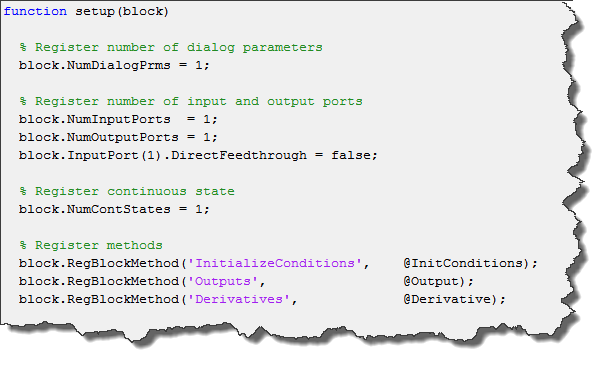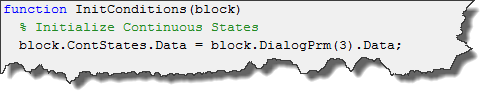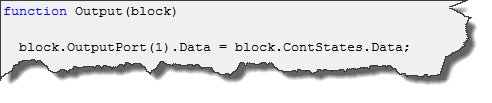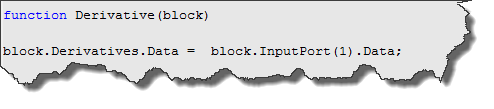# Creating Your Own Block With Continuous States (MATLAB S-Function)

In a previous post, I showed an example of a MATLAB S-Function with a discrete state

This week, we continue in the same direction and implement an S-Function with a continuous state.

Continuous States

To begin, let's go back to the question posted on MATLAB Answers by K E:

What are continuous and discrete states in Simulink?

and see what Kaustubha Govind mentionned about continuous states:

With continuous states however, Simulink asks the block to provide a derivative (dx/dt) of the state in the Derivatives() method and uses its ODE solver to compute the integral of dx/dt to obtain 'x'. This 'x' can then be accessed in the Outputs() function. For example, to implement an Integrator block...

Let's stop here and see how to implement an Integrator block step by step.

Setup

In an S-Function, the continuous states are declared in the setup method:Continuous states can not be hidden, like discrete states. There are many reason for this, for example, they are used by the Simulink solver to compute steps taken by the variable time-step solver.

For details on the DirectFeedthrough flag, look at the previous post about discrete states.

Initialize Conditions

Like the discrete state, you need to initialize continuous states:Outputs

In the Outputs method, you can read the value of the continuous state and assign it to the output port:Derivatives

Here is the most important part. When you have continuous states, you are not supposed to write to block.ContStates.Data, except for initialization. Instead, you specify the derivative of the states and write that to block.Derivatives.Data. For an Integrator block, the input is the derivative of the output:The next time the output method will be called, the solver will have integrated the value specified in block.Derivatives.Data and stored the result in block.ContStates.Data.

Conclusion

The important thing to realize about continuous states is that the block provides the derivative of the states to the solver. The solver takes care of the integration and gives back to the block the result of the integration.

For an example like that, look at the demo model msfcndemo_limintm.mdl